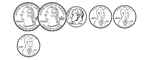63 Cents

Groups of change with totals from 1 to 100 cents using the least amount of coins.64 Cents

Groups of change with totals from 1 to 100 cents using the least amount of coins.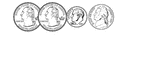65 Cents

Groups of change with totals from 1 to 100 cents using the least amount of coins.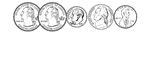66 Cents

Groups of change with totals from 1 to 100 cents using the least amount of coins.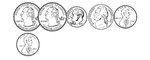67 Cents

Groups of change with totals from 1 to 100 cents using the least amount of coins.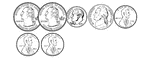68 Cents

Groups of change with totals from 1 to 100 cents using the least amount of coins.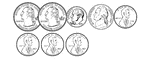69 Cents

Groups of change with totals from 1 to 100 cents using the least amount of coins.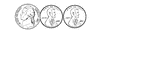7 Cents

Groups of change with totals from 1 to 100 cents using the least amount of coins.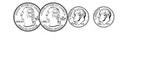70 Cents

Groups of change with totals from 1 to 100 cents using the least amount of coins.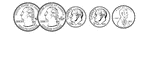71 Cents

Groups of change with totals from 1 to 100 cents using the least amount of coins.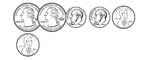72 Cents

Groups of change with totals from 1 to 100 cents using the least amount of coins.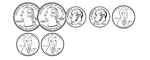73 Cents

Groups of change with totals from 1 to 100 cents using the least amount of coins.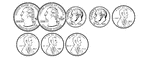74 Cents

Groups of change with totals from 1 to 100 cents using the least amount of coins.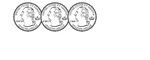75 Cents

Groups of change with totals from 1 to 100 cents using the least amount of coins.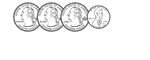76 Cents

Groups of change with totals from 1 to 100 cents using the least amount of coins.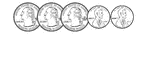77 Cents

Groups of change with totals from 1 to 100 cents using the least amount of coins.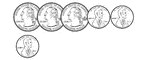78 Cents

Groups of change with totals from 1 to 100 cents using the least amount of coins.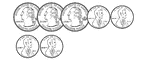79 Cents

Groups of change with totals from 1 to 100 cents using the least amount of coins.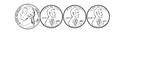8 Cents

Groups of change with totals from 1 to 100 cents using the least amount of coins.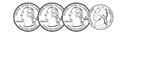80 Cents

Groups of change with totals from 1 to 100 cents using the least amount of coins.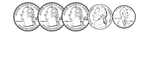81 Cents

Groups of change with totals from 1 to 100 cents using the least amount of coins.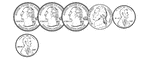82 Cents

Groups of change with totals from 1 to 100 cents using the least amount of coins.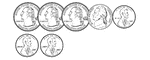83 Cents

Groups of change with totals from 1 to 100 cents using the least amount of coins.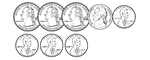84 Cents

Groups of change with totals from 1 to 100 cents using the least amount of coins.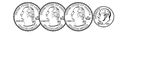85 Cents

Groups of change with totals from 1 to 100 cents using the least amount of coins.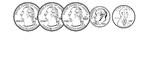86 Cents

Groups of change with totals from 1 to 100 cents using the least amount of coins.87 Cents

Groups of change with totals from 1 to 100 cents using the least amount of coins.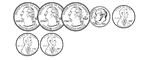88 Cents

Groups of change with totals from 1 to 100 cents using the least amount of coins.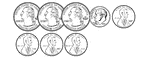89 Cents

Groups of change with totals from 1 to 100 cents using the least amount of coins.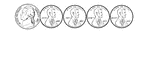9 Cents

Groups of change with totals from 1 to 100 cents using the least amount of coins.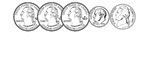90 Cents

Groups of change with totals from 1 to 100 cents using the least amount of coins.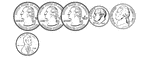91 Cents

Groups of change with totals from 1 to 100 cents using the least amount of coins.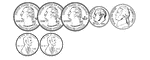92 Cents

Groups of change with totals from 1 to 100 cents using the least amount of coins.93 Cents

Groups of change with totals from 1 to 100 cents using the least amount of coins.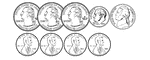94 Cents

Groups of change with totals from 1 to 100 cents using the least amount of coins.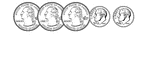95 Cents

Groups of change with totals from 1 to 100 cents using the least amount of coins.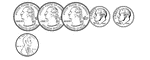96 Cents

Groups of change with totals from 1 to 100 cents using the least amount of coins.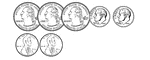97 Cents

Groups of change with totals from 1 to 100 cents using the least amount of coins.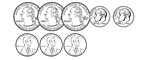98 Cents

Groups of change with totals from 1 to 100 cents using the least amount of coins.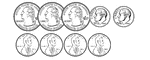99 Cents

Groups of change with totals from 1 to 100 cents using the least amount of coins.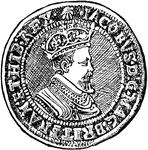Double Crown

A gold coin of the value of 10 or 11 shillings, current in England in the seventeenth century. It was…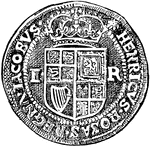Double Crown

A gold coin of the value of 10 or 11 shillings, current in England in the seventeenth century. It was…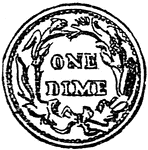Dime

A silver coin of the United States, of the value of ten cents.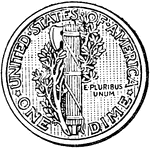Mercury Dime

Reverse side of American ten cent piece with fasces and olive branch (1916-1945).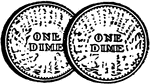2 Dimes

Illustration showing the back side of two dimes.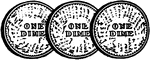3 Dimes

Illustration showing the back side of three dimes.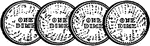4 Dimes

Illustration showing the back side of four dimes.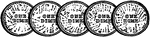5 Dimes

Illustration showing the back side of five dimes.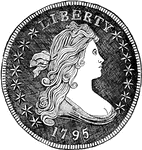United States Silver Dollar

The back of a United States Silver Dollar. Worth 100 cents.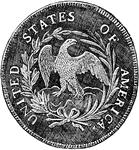United States Silver Dollar

The back of a United States Silver Dollar. Worth 100 cents.